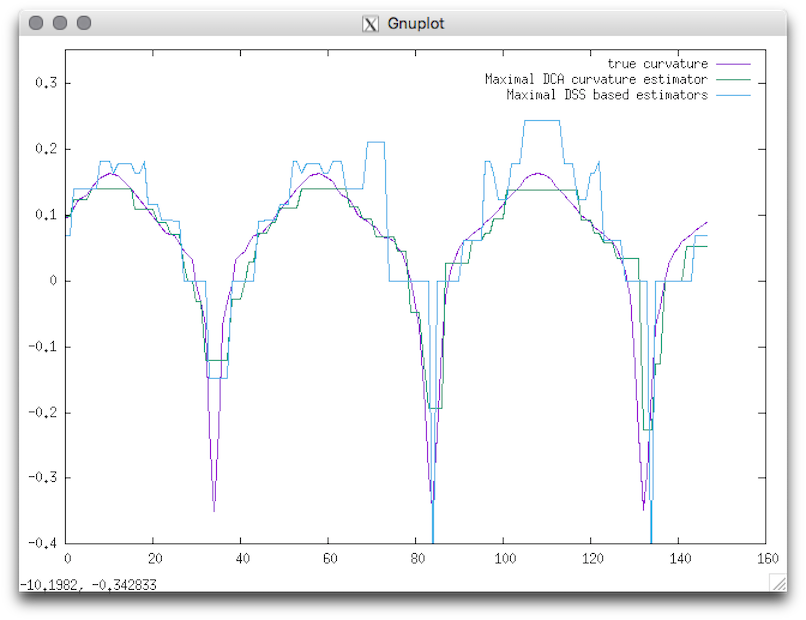DGtalTools  1.3.beta
2dLocalEstimators

Compares local estimators on implicit shapes using DGtal library.

Usage: 2dlocalEstimators –output <output> –shape <shapeName> [required parameters] –estimators <binaryWord> –properties <binaryWord>

Below are the different available families of estimators:

• True estimators
• Maximal DSS based estimators
• Maximal DCA based estimators
• Binomial convolver based estimators
• Integral Invariants based estimators

The i-th family of estimators is enabled if the i-th character of the binary word is not 0. The default binary word is '10000'. This means that the first family of estimators, ie. true estimators, is enabled, whereas the next ones are disabled. Below are the different available properties:

• Tangent
• Curvature

Allowed options are :

-h,--help Print this help message and exit
-l,--list List all available shapes
-o,--output TEXT REQUIRED Output
-s,--shape TEXT REQUIRED Shape name
-K,--kernelradius FLOAT=2.35162e-314 Radius of the convolution kernel (Integral invariants estimators)
--alpha FLOAT=0.333333 Alpha parameter for Integral Invariant computation
-A,--axis1 FLOAT Half big axis of the shape (ellipse)
-a,--axis2 FLOAT Half small axis of the shape (ellipse)
-r,--smallradius FLOAT=5 Small radius of the shape
-v,--varsmallradius FLOAT=5 Variable small radius of the shape
-k UINT=3 Number of branches or corners the shape (default 3)
--phi FLOAT=0 Phase of the shape (in radian)
-w,--width FLOAT=10 Width of the shape
-p,--power FLOAT=2 Power of the metric
-x,--center_x FLOAT=0 x-coordinate of the shape center
-y,--center_y FLOAT=0 y-coordinate of the shape center
-g,--gridstep FLOAT=1 Gridstep for the digitization
-n,--noise FLOAT=0 Level of noise to perturb the shape
--properties TEXT=11 the i-th property is disabled iff there is a 0 at position i
-e,--estimators TEXT=1000 the i-th estimator is disabled iff there is a 0 at position i
-E,--exportShape TEXT Exports the contour of the source shape as a sequence of discrete points (.sdp)
--lambda BOOLEAN=0 Use the shape to get a better approximation of the surface (optional)

Example: With this tool you can easely compare several estimator with the real value:

$2dlocalEstimators --output curvature --shape flower --radius 15 -v 5 --gridstep 1 --estimators 11100 --properties 01 You can display the result by using gnuplot:$ gnuplot
gnuplot> plot [][-0.4:0.35] 'curvature_True_curvature.dat' w lines title "true curvature" , 'curvature_MDCA_curvature.dat' w lines title "Maximal DCA curvature estimator", 'curvature_MDSSl_curvature.dat' w lines title "Maximal DSS based estimators"

You should obtain such a graph:Resulting visualization.